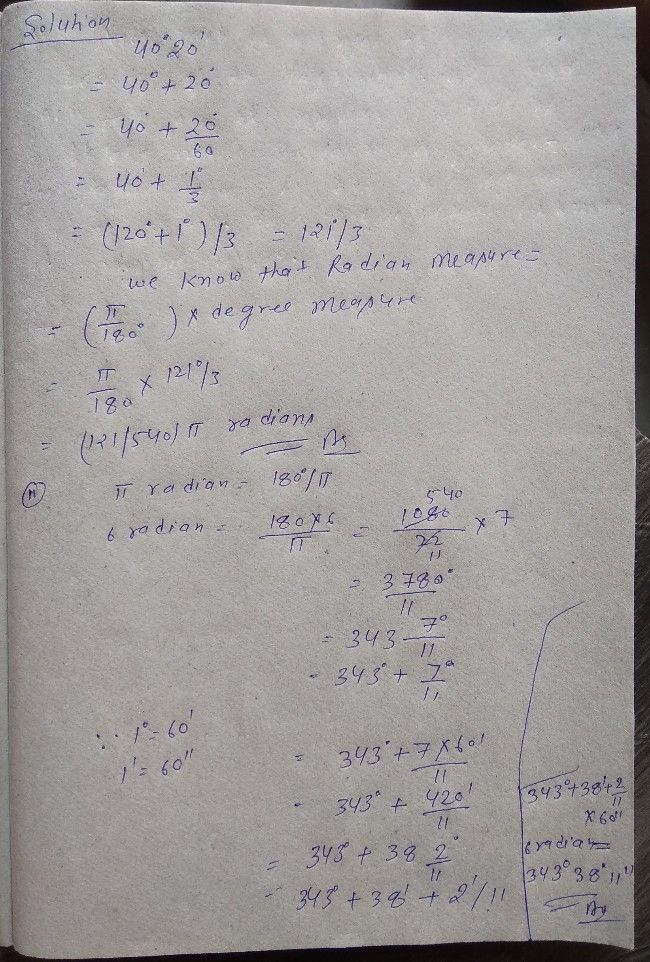Symbol
Problem$1sx3.1$ $1.$ Convert $40^{°}20^{'}$ into radian measure. $2$ Convert $6$ radians into degree measure. $3$ Find the radius of the circle in which a central angle of $609$ intercepts an $a$
10th-13th grade
Trigonometry
Search count: 107
SolutionQanda teacher - LaxmiStudent
thanks sir